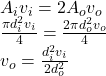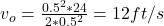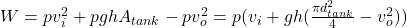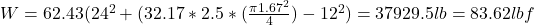## water in the tank is 2.5 ft. Water enters the tank through a top port of diameter 6 in. at a velocity of 24 ft/s. The water leaves the tank

Question

water in the tank is 2.5 ft. Water enters the tank through a top port of diameter 6 in. at a velocity of 24 ft/s. The water leaves the tank through two exit ports each with a diameter of 6 in. If the scale shows a reading of 585 lbf, calculate the weight of the tank when it is empty

in progress 0
6 months 2021-08-03T14:23:53+00:00 1 Answers 3 views 0

## Answers ( )

The weight of the tank when it is empty is 501.38 lbf

Explanation:

The velocity of outlet is equal to:Where

vi = 24 ft/s

di = 6 in = 0.5 ft

do = 6 in = 0.5 ftThe total weight of the water excluding the tank weight is equal to:p = 62.43 lb/ft³

dtank = 20 in = 1.67 ft

Replacing:The weight of the tank is:

Wtank = 585 – 83.62 = 501.38 lbf